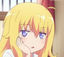top of pageSearch
•Jian Gao

# Bregman Learning for Generative Adversarial Networks

Updated: Mar 4, 2018

A game theoretic framework for deep generative learningIn this paper we develop a game theoretic learning framework for deep generative models. Firstly, the problem of minimizing the dissimilarity between the generator distribution and real data is introduced based on f-divergence. Secondly, the optimization problem is transformed into a zero-sum game with two adversarial players, and the existence of Nash equilibrium is established in the quasi-concave-convex case under suitable conditions. Thirdly, a general Bregman-based learning algorithm is proposed to find the Nash equilibria. The algorithm is proved to have a doubly logarithmic convergence time with respect to the precision of the minimax value in potential convex games. Lastly, our methodology is implemented in three application scenarios and compared with several existing optimization algorithms. Both qualitative and quantitative evaluation show that the generative model trained by our algorithm has the state-of-art performance.

• Contribution of the present paper

First, we have developed a game theoretic learning framework to train generative models. The objective of the generator is to ”cheat” the discriminator by maximizing its error, which leads to a minimax problem. Putting it in the context of game theory, this problem is transformed into a zero-sum game where the generator and discriminator are two adversarial players. This is also called minimax robust game.

Then, we look for the solution for both sides and proved the existence of Nash equilibria under quasi-concave-convex assumptions. Since quasi-convexity is a weaker property than convexity, the result allows to extend earlier works to including a class of non-convex situations. Once the existence of equilibrium is guaranteed, the next step is to find the equilibrium through iterative learning procedure or closed-form expressions.When the underlying payoff function is concave/convex, we use Lagrangian and Bregman approach to derive the model-based learning procedure. The algorithm is shown to be double exponentially fast and converges very quickly to the Nash equilibrium. When the payoff is not convex/concave, a modified algorithm that stabilizes the learning process is used. The Bregman learning algorithm is a general configurable approach. We will show that the classical gradient based methods such as stochastic gradient descent (SGD) and Momentum are two special cases under our scheme.

Finally, we evaluate the performance of our approach in three application scenarios, and compare it with several existing algorithms in this field. Both qualitative and quantitative results show that the generative model trained by our algorithm has state-of-the-art performance.Comparison of seven stochastic optimization algorithms

This paper has been accepted by Chinese Control and Decision Conference (CCDC) and will be published in June 2018.